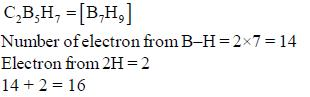Courses

# Main Group Elements

## 20 Questions MCQ Test GATE Chemistry Mock Test Series | Main Group Elements

Description
This mock test of Main Group Elements for Chemistry helps you for every Chemistry entrance exam. This contains 20 Multiple Choice Questions for Chemistry Main Group Elements (mcq) to study with solutions a complete question bank. The solved questions answers in this Main Group Elements quiz give you a good mix of easy questions and tough questions. Chemistry students definitely take this Main Group Elements exercise for a better result in the exam. You can find other Main Group Elements extra questions, long questions & short questions for Chemistry on EduRev as well by searching above.
QUESTION: 1

### Select the energy band which represent the electrical conductivity in direction parallel to chain in graphite

Solution:

Graphite behave as semimetal in direction parallel to chain. Second band represent the band structure of semimetal. Hence, correct option is (b)

QUESTION: 2

### KMg3(OH)2Si3AlO10 is an example of

Solution: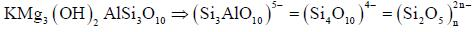QUESTION: 3

### Correct order of metal ion regarding logβ value for [M-crown-6]+ in gas phase is

Solution:

In gas phase, ion tends to form stable complex with ligand having more contact point (donor atom) rather than more preference to cavity size. Hence, Na+ due to high charge density than K+ favour crown-6 more than K+ in gas phase. Correct option is (b)

QUESTION: 4

Reaction of S4N4 with metallic potassium or sodium leads to formation of

Solution: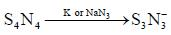QUESTION: 5

Reaction of B2H6 with Na/Hg leads to formation of​

Solution: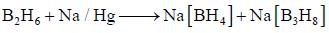QUESTION: 6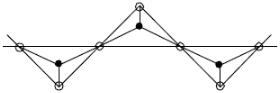The formula and type of above borate is

Solution:

In the above diagram, two oxygen atom is shared hence it is metaborate.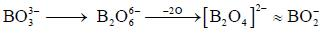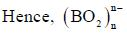*Answer can only contain numeric values
QUESTION: 7

Number of cluster electron pair in [In4Bi5]3- is ________

Solution: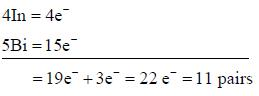*Answer can only contain numeric values
QUESTION: 8

The number of Banana bond in B5H9 is _____

Solution:

STYX code of B5H9 = 4120 where S represents number of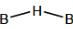bond also called Banana Bond.

*Answer can only contain numeric values
QUESTION: 9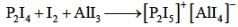31P NMR spectrum of cation shows _____doublet of equal intensity

Solution: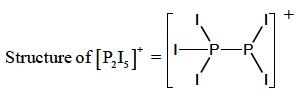*Answer can only contain numeric values
QUESTION: 10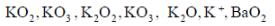Number of species paramagnetic in nature is/are______

Solution: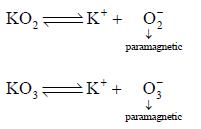*Answer can only contain numeric values
QUESTION: 11

Hydrolysis of P2X4 produces an acid which have basicity _________

Solution: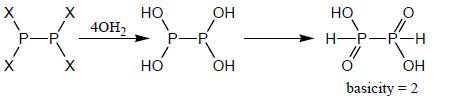QUESTION: 12

Electrophilic substitution reaction of terminal H atom attached to B in closo-1, 2 C2B10H12 follow the sequence

Solution:

Terminal H atom attached to Boron undergo facile electrophilic substitution and sequence of reactivity follow the sequence of negative charge density on B–Ht group.
More the distance from carbon = more the reactivity towards nucleophile.

QUESTION: 13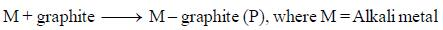The correct statement regarding P is/are
(I) electrical conductivity decreases with increase in temperature
(II) M-graphite compounds are coloured.
(III) Mechanism of conductivity in M-graphite complexes are similar to conductivity in n-type semiconductors.
(IV) In product planarity and delocalization of layers are retained.

Solution:

Metal graphite complexes has conductivity like Metals and their conductivity decreases with increase in temperature.
These complexes have excess electron in valence band. Hence, their conductivity is due to migration of free electron.

QUESTION: 14

Match Column-A (species) with Column-B (properties related to species)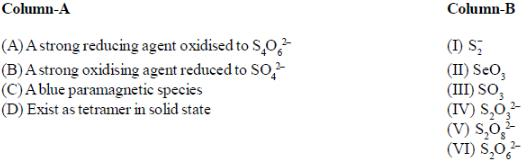Solution: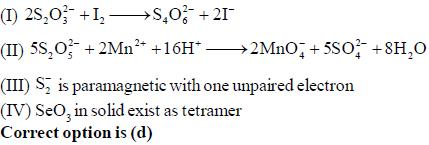QUESTION: 15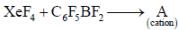Correct statement regarding A is/are
(1) It has T-shaped structure
(2) 129Xe NMR spectrum show a triplet
(3) 19F NMR spectrum show four signal
(4) It has TBP geometry

Solution: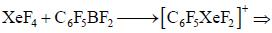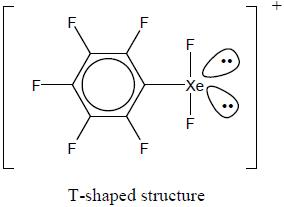QUESTION: 16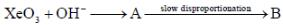Correct statement regarding A and B are
(1) A is very strong oxidising agent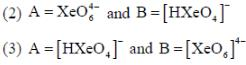(4) B is strong oxidising agent.

Solution: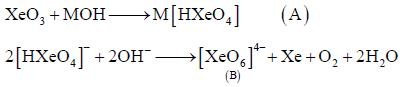QUESTION: 17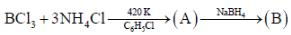In the above reaction A and B respectively are

Solution: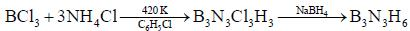QUESTION: 18

Correct statement regarding C60 is/are
(1) It has Ih point group
(2) Its satisfies IPR
(3) It has two kind of C–C bond length
(4) It is more reactive than graphite

Solution:

No pentagons are adjacent to each other in C60 and also it has two kinds of bond length, one between two hexagon and one between one hexagon and pentagon.

QUESTION: 19

CH3Cl + Si + HCI → (A)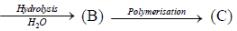The (C) is

Solution: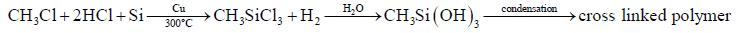*Answer can only contain numeric values
QUESTION: 20

Number of framework electron in carbonanes C2B5H7 is ________

Solution: